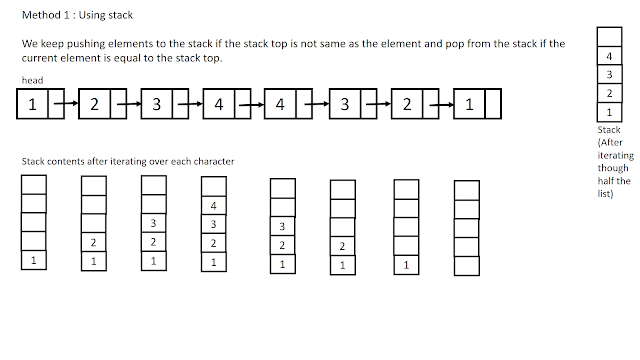### Check if a Linked List is Palindrome

In this post, We will discuss a method to check if a given string or number represented in the form Linked List is a Palindrome. First, let's see the definition of a Palindrome, A word, phrase or a sequence that reads the same backwards as forwards is called a Palindrome. eg. "MADAM", 11100111, "NURSES RUN".

Questions on palindrome, anagrams are very common in coding interviews and also very easy to solve if you have solved them before. There are a lot of methods to check If a string or a number is a palindrome or not. In this post, we will use the second method i.e pointers

First Approach: Using Stack

In this method we iterate over the string and for each character we compare the current character is same as that of character at the stack top if yes then we pop the element from the stack top else we push the current character onto the stack, after iterating over the string if the stack is empty then we can declare that the given string is a palindrome else the given string is not a palindrome.

IMP: If the length of the string is odd then we do not push the middle character to the stack.

This method is efficient as we iterate through the linked list only once but it uses extra space in form of a stack.

Algorithm:

1. Create an empty Stack
2. Push the head node onto the stack
3. While the last node of the linked list is not reached do the following actions
1. If the contents of the current node and the node at the stack top are same then pop the element from the stack
2. else push the current node on the stack
4. If we have traversed through the Linked List and the stack is empty then we Return that the String is a palindrome.Second Approach: Using 2 pointers

In this method, we use 2 pointers left and right and we recursively traverse the linked list. In this method right node points to the tail of the linked list and left node will point to the head of the linked list and if the characters are same at both the node then we move left node forward and right node backward recursively. We return the result in each recursive call.

Algorithm:

1. Initialize right and left pointers to head node, make left node as static node pointer
2. Move the right node to the end of the linked list recursively
3. As soon as the right node reaches the end of the linked list do the following actions
1. Compare the data field of left and right nodes if they are same then move the left node forward and return true
Let's have a look at the code

### Sample input and output to check the program

You might also be interested in

### Infix to Prefix conversion using Stack

This post is about conversion of Infix expression to Prefix conversion. For this conversion we take help of stack data structure, we need to push and pop the operators in and out of the stack.

Infix expressions are the expressions that we normally use,eg. 5+6-7; a+b*c etc. Prefix expressions are the expressions in which the 2 operands are preceded by the operator eg. -+567 , +a*bc etc.

This method is very similar to the method that we used to convert Infix to Postfix but the only difference is that here we need to reverse the input string before conversion and then reverse the final output string before displaying it.

NOTE: This changes one thing that is instead of encountering the opening bracket we now first encounter the closing bracket and we make changes accordingly in our code.

So, to convert an infix expression to a prefix expression we follow the below steps
(we have 2 string, 1st is the input infix expression string 2nd is the output string which is empty initially)

We first revers…

Hashing is a technique used for storing , searching and removing elements in almost constant time. Hashing is done with help of a hash function that generates index for a given input, then this index can be used to search the elements, store an element, or remove that element from that index.

A hash function is a function that is used to map the data elements to their position in the data structure used. For example if we use an array to store the integer elements then the hash function will generate position for each element so that searching, storing and removing operation on the array can be done in constant time that is independent of the number of elements in the array. For better look at the example below.

now we face a problem if for 2 numbers same position is generated example consider elements 1 and 14

1 % 13 = 1

14 % 13 = 1

so when we get 1 we store it at the first position, but when we get 14 we see that the position 1 is already taken, this is a case of collision.

Inorder…